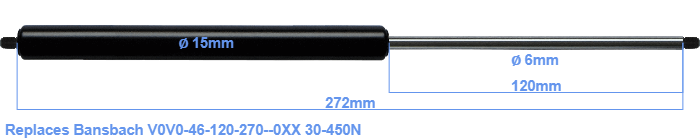# Replacement for Bansbach V0V0-46-120-270–0XX 30-450N

\$26.92

Replacement gas spring for the Bansbach V0V0-46-120-270--0XX 30-450 Newton. Thread: M5. Brand: Hahn.
 Force Choose an option30 Newton40 Newton50 Newton60 Newton80 Newton100 Newton120 Newton140 Newton150 Newton160 Newton180 Newton200 Newton220 Newton240 Newton250 Newton260 Newton280 Newton300 Newton320 Newton340 Newton350 Newton360 Newton380 Newton400 Newton420 Newton440 Newton450 NewtonClearThis gas spring has a cylinder diameter of 15 mm. The cross section of the rod is 6 mm. The stroke of the gas spring is 120 millimeter. The total length of this replacement is 272 millimeter. This is the total distance between the centers of the two mounting parts. The length from thread to thread (exlcuding the mounting parts) is 272 millimeter. This replacement gas spring has a force of 30-450 Newton. This is not an original gas spring from Bansbach, but a so called replacement gas spring. This is a Stabilus Industry Line gas spring. Nevertheless, the force and dimensions equal.